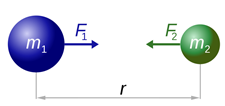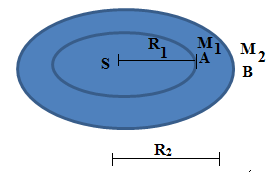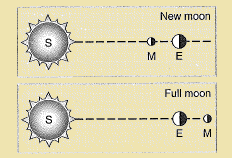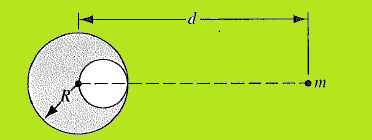Click to Chat

1800-1023-196

+91-120-4616500

CART 0

• 0

MY CART (5)

Use Coupon: CART20 and get 20% off on all online Study Material

ITEM
DETAILS
MRP
DISCOUNT
FINAL PRICE
Total Price: Rs.

There are no items in this cart.
Continue Shopping• Complete Physics Course - Class 11
• OFFERED PRICE: Rs. 2,968
• View Details

Newton’s Law of Gravitation

Table of Content

Statement

Definition of G

Units and Value of G

Derivation of Newton’s Law of Gravitation using Kepler’s third Law and Vice-Versa

Conceptual Questions

Solved Problems

Related Resources

Statement“Every particle of matter in this universe attracts every other particle with a forcer which varies directly as the product of masses of two particles and inversely as the square of the distance between them.”

The force of attraction between any two bodies in the universe is known as the force of gravitation. This force is mutual and acts along the line joining the centers of two bodies.

Consider two bodies of masses m1 and m2 as shown in below figure. Let r be the distance between their centers. Let F be the magnitude of the force of attraction  between them. According to the law of gravitation,

(a) F∝ m1m2

(b) F∝1/r2

Combining the above two factors, we get,

F∝m1m2/r2

or

F = G [m1m2/r2]

Here G is a constant of proportionality. This constant has the same value everywhere and is known as universal constant of gravitation or gravitational constant or constant of universal gravitation.Definition of G

Let m1 = m2 = 1 unit r = 1 unit

Then, from equation (3), we get,

F = G (11/12) = G

or G = F

Thus, the gravitational constant may be defined as the magnitude of force of attraction between two bodies each of unit mass and separated by a unit distance from each other.

Units and Value of G

(a) C.G.S System:-

Unit:- dyne cm2 g-2

Value:- 6.6710 -8 dyne-cm2 gram-2

(b) SI System:-

Unit:- N m2 kg-2

Value:- 6.6710 -11 N m2 kg-2

Dimensional formula of G:-

G = Fr2/Mm =[MLT-2][L2]/[M2] = [M-1L3T-2]

Derivation of Newton’s Law of Gravitation using Kepler’s third Law and Vice-Versa

Any of these two laws can be derived by using the second one.

(a) Derivation of Newton’s law of gravitation using Kepler’s law:-By using some approximation that the orbit of a planet be assumed to be circular instead of elliptical, we can deduce Newton’s law of gravitation from Kepler’s law.

Consider two planets A and B having masses M1 and M2 revolving around the sun, in circular orbits, of radii R1 and R2 with angular velocities ω1 and ω2 respectively as shown in the below figure. Centripetal forces F1 and F2 acting on them are,

F1  = M1R1ω12

F2  = M2R2ω22

So, F1/F2 = M1R1ω12 / M2R2ω22

= M1R1/M2R2 (2π/T1)2 (T2/2π)2

Here T1 and T2 are the time periods of revolution of two planets.

F1/F2 = M1R1/M2R2 (T22/T12)

According to Kepler’s third law, T22/T12 = R23/R13

Substituting for T22 /T12,  F1/F2 = M1/M2 R1/R2R23/R13 = (M1/M2) (R22/R12)

Or, F∝M/R2

That is force of attraction varies directly as mass of body and inversely as the square of the distance.

or   F = K (M/R2)  If K = Gm

Here m is the mass of the sun.

F = GMm/R2

Which is Newton’s law of gravitation.

(b) Derivation of Kepler’s third law using Newton’s law of gravitation:-

For simplicity let us assume that the earth revolves round the sun in circular orbit. Let R be the radius of the orbit, T and  be the time period and velocity of the earth around the sun respectively.

T = 2πR/

or, T2 = 4π2R2/2 = 4π2R3/2R          …... (1)

If M and m be masses of the sun and earth respectively, we have,

mv2/R = G Mm/R2

Thus, v2R = GM              …... (2)

Substituting equation (2) in equation (1), we get,

T2 = 4π2R3/GM = KR3

Here K =  4π2/GM = a constant

Therefore, T2 ∝ R3

This is Kepler’s third law.The gravitational force is an attractive force.

The gravitational force between two particles does not depend on the medium.

The gravitational force between two particles is along the straight line joining the particles (called line of centers).Question 1:-

The atmosphere is held to the earth by:

(a) Winds

(b) gravity

(c) clouds

(d) the gravitation of the earth

Question 2:-

Gravitational forces are:

(a) always attractive

(b) always repulsive

(c) sometimes attractive and sometimes repulsive

(d) none

Question 3:-

A ball is thrown up vertically in air. As it rises there is an increase in its

(a) Potential energy

(b) Kinetic energy

(c) Acceleration

(d) None of them

Question 4:-

Which of the following is the evidence to show that there must be a  force acting on earth and directed towards the sun:

(a) deviation of the falling bodies towards east

(b) revolution of earth around the sun

(c) phenomena of day and night

(d) apparent motion of sun round the earthQ.1

Q.2

Q.3

Q.4

b

a

a

b

Conceptual Questions

Question 1:-

Would you expect the toatl energy of the solar system to be constant? The total angular momentum? Explain your answers.

Solution:-

The total energy of the planet orbiting around the Sun is constant.

It is given by

E = GMm/r

Here Mass of the Sun, M Mass of the planet, m  Gravitational constant, G Orbital radius of the planet about the Sun, r.

From the law of conservation of energy, energy cannot be created nor can be destroyed. The total energy of the solar system is equal to the sum of the total energy of the planets around the Sun, the corresponding orbiting moon around the corresponding planets. Thus, the total energy of the solar system is constant.

Angular momentum is the rate of change of angular momentum.

There is no external torque acting on the solar system. This is due to the fact that the motions of the planets are not disturbed. Thus, the total angular momentum of the solar system is constant.

___________________________________________________________________________________

Question 2:-

A satellite in Earth orbit experiences a small drag force as it starts to center the Earth’s atmosphere. What happens to its speed? (Be careful!)

Solution:-

It is given that a satellite in Earth’s orbit experiences a small drag force as it starts to enter the Earth’s atmosphere. As the drag force is small, the satellite enters the Earth’s atmosphere with much large force. This means it accelerates at a large value. It is known that an object falls towards the Earth at free-fall acceleration of the Earth. Thus, the satellite will uniformly accelerate and its speed will be enormous.

__________________________________________________________________________________________

Question 3:-

Partucularly large tides, called spring tides, occur at full moon and at new moon, when the configurations of the Sun, Earth, and Moon are shown in below figure. From the figure you might conclude (incorrectly!) that the tidal effects of the Sun and of the Moon tend to add at new  moon but cancel at full moon. Instead, they add at both these configurations. Explain Why.Solution:-

The large tides are mainly due to the gravitational attraction of the Sun, the Earth and the Moon. The main contribution comes from the gravitational attraction between the Earth and the Moon. As it is given spring tides are due to the configurations of the Sun, Earth and Moon in the straight line. It does depend on the location in the straight line. So, they never cancel each other. Instead the mutual gravitational forces are added and large tides are produced.

______________________________________________________________________________________

Solved Problems

Problem 1:-

A spherical hollow is made in a lead sphere of radius R, such that its surface touches the outside surface of the lead sphere and passes through its center (see below figure). The mass of the sphere before hollowing was M. With what force, according to the law of universal gravitation, will the hollowed lead sphere attract a small sphere of mass m, which lies at a distance d from the center of the lead sphere on the straight line connecting the centers of the spheres and of the follow?  [1946, Olympic, Moscow State University]Concept:-

The force of gravity on the small sphere of mass m due to the lead sphere after it is made hollow is equal to the force of gravity from a solid lead sphere minus the force which would have been contributed by the smaller lead sphere which would have filled the hole.

Solution:-

From the Newton’s law of universal gravitation, the force of attraction between the lead sphere and the small sphere is

F1 = GMm/d2

Here, gravitational constant is G, total mass of the lead sphere is M, mass of the small sphere is m and distance between the centers of the spheres is d.

So, we need to know about the size and mass of the lead which was removed to make the hole.

The density of the lead is given by

ρ = M/[(4/3)(πR3)]

The spherical sphere has a radius of R/2.

If the density is constant, then the mass of the hole will be the density times the volume of the hollow sphere. Thus, the mass of the hollow sphere is

Mh = V

= [M/[(4/3)(πR3)]] [4/3 {π (R/2)3}]

= M/8

The hole is closer to the small sphere.  This means the center of the hole is d-(R/2) away from the small sphere.

Now, the gravitational force between the hollow sphere and the small sphere is

F2 = GMhm/(d-R/2)2

= [G(M/8)m]/(d-R/2)2

The force of attraction with which the lead sphere after it is made hollow and the small sphere is equal to the force of the whole lead sphere before it is made hollow minus the force of the hollow lead sphere. The corresponding force is

F = F1 – F2

= [GMm /d2] – [[G (M/8) m]/[d-R/2]2]

= GMm [1/d2 – 1/2(d-R)2]

= [GMm/2d2 (d-R)2] [d2 – 2R2 – 4dR]

Therefore, the required force from the lead sphere which attract the small sphere is

[GMm/2d2 (d-R)2] [d2 – 2R2 – 4dR]

____________________________________________________________________________________

Problem 2:-

A certain triple-star system consists of two stars, each of mass m, revolving about a central star; mass M, in the same circular orbit. The two stars stay at opposite ends of a diameter of the circular orbit; see below figure. Derive an expression for the period of revolution of the stars; the radius of the orbit is r.Concept:-

The gravitational force due to the two stars to the central star is

F1 = GMm/r2 + GMm/r2

= 2 GMm/r2

Here, gravitational constant is G, mass of the central star is M, mass of the orbiting stars is m and radius of the orbit is r.

Also, the gravitational force between the orbiting stars is,

F2 = Gmm/(2r)2

= Gm2/4r2

The net gravitational force acting on the stars is equal to the sum of the gravitational forces acting on the system. It is given as,

Fnet = F1+F2

= 2 GMm/r2 + Gm2/4r2

= Gm/r2 [(2M)+m/4]

The centripetal force that makes the stars to orbit around the central star is

Fc = 4π2mr/T2

Here, period of the stars around the central star is T.

Solution:-

The centripetal force is provided by the net gravitational force acting on the system of stars. Thus,

Fnet = Fc

Substitute Gm/r2 [(2M)+m/4] for Fnet  and 4π2mr/T2 for Fc in the above equation.

Fnet = Fc

Gm/r2 [(2M)+m/4] = 4π2mr/T2

So, T = √16π2r3/[G(8M+m)]

Therefore, the expression for the period of revolution of the stars is √16π2r3/[G(8M+m)].

____________________________________________________________________________________________

Problem 3:-

In 3001: The Final Odyssey, Authur C. Clarke writes of a tower that stretches from the Earth’s equator to geosynchronous orbit. (a) The hero, Frank Poole, finds himself in the tower and estimates the acceleration of free fall at his altitude to be g/2. Taking into acount rotational motion, what is pole’s altitude? (b) Calculate the work necessary to raise a 100-kg mass from the surface of the Earth up through the tower to the geosynchronous altitude. Compare your result to the energy expenditure of a rocket that can do the same thing today. (Hint: Assume that the rotational correction is small, and solve iteratively.)

Solution:-

The gravitation acceleration on the surface of the neutron star is equal to the free-fall acceleration on its surface. Any object falling on the neutron star will accelerate at its free-fall acceleration. As the object fell from rest, its final velocity is equal to zero. The mass of the Sun is about 0.991030 kg.

Given data:

Mass of the neutron star, MNeutron = 1.991030 kg.

Gravitation constant, G = 6.6710-11 N.m2/Kg2

RNeutron = 10 km = 10,000 m

Distance moved by the object on the neutron star, x = 1.20 m

Initial speed of the object, v0x = 0

(a) The gravitation acceleration at the surface of the neutron stars is equal to its free-acceleration on its surface.

Thus, the gravitation acceleration is

gNeutron = GMNeutron / r2Neutron

=  (6.6710-11 N.m2/Kg2) (1.99  1030 kg)/(10,000 m)2

= 1.32733 1012 m/s2

Rounding off to three significant figures, the gravitational acceleration at the surface of the neutron star is1.32733 1012 m/s2 .

(b) Using the kinetic equation vx2 = v0x2 - 2gNeutron  in one-dimension, the final velocity of the object on the neutron stars is

vx = √v0x2 - 2gNeutron (-x)

The negative sign shows that the displacement of the object is moving towards the center of the neutron star.

Thus,

vx = √0 - 2gNeutron (x)

= √2( 1.33 1012 m/s2) (1.20 m)

= 1.7867106 m/s

Rounding off to three significant figures, the object will be moving at the speed of 1.7867106 when it feel from rest through a distance of 1.20 m on the neutron star.

______________________________________________________________________________

Problem 4:-

Two Lead balls of radius 10 cm and 1 cm are placed with their centres 1 metre apart. Calculate the force of attraction between them. The density of Lead is 5.51 × 103 kg/m3.

Solution:

Density of balls, ρ = 5.51× 103 kg/m3

Force of attraction between them, F = Gm1m2/r2

where m1 = 4/3Πr23 × ρ = 5.51 × 103 × 4/3 Π10-3 ≈ 23 kg

and m2 = 4/3Πr23 × ρ ≈ 23 × 10-3 kg

G = 6.67 × 10-11 Nm2 kg-2

Therefore, F = 6.67 × 10-11 × 21 × 21 × 10-3N = 3.5 × 10-11 N

Related Resources

You might like to refer Gravitational Field and Intensity

For getting an idea of the type of questions asked, refer the Sample Papers of Previous Years.### Course Features

• 101 Video Lectures
• Revision Notes
• Previous Year Papers
• Mind Map
• Study Planner
• NCERT Solutions
• Discussion Forum
• Test paper with Video Solution